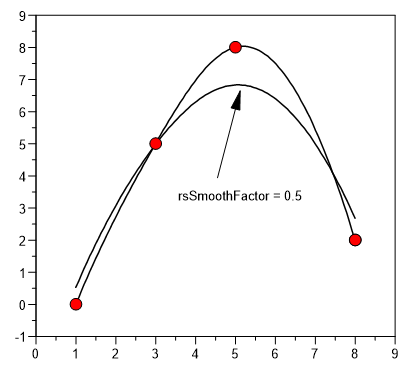# fspline¶

fspline computes a fit spline for a given x- and y-vector.

`rmXY = fspline(rvX, rvY)`
`rmXY = fspline(rvX, rvY, rsSmoothFactor)`
`rmXY = fspline(rvX, rvY, rsSmoothFactor, nPoints)`

Return Value

The return value rmXY is a real matrix with two columns. The first column contains the x coordinates of the spline and the second column contains the y coordinates of the spline.

Parameters

`rvX`

rvX is a real vector with at least 3 elements in increasing order.

`rvY`

rvY is a real vector with at least 3 elememts.

`rsSmoothFactor`

rsSmoothFactor is an integer equal to or greater than 0.0. For 0.0 the spline will cross all data points.

`nPoints`

nPoints is the number of points of rmXY.

Example```x = [1,3,5,8]
y = [0,5,8,2]
h = plot(x,y)
xy = fspline(x,y,0,100)
plot(xy[;1],xy[;2],h)
xy = fspline(x,y,0.5,100)
plot(xy[;1],xy[;2],h)
```